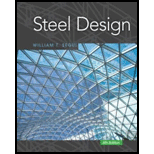# a . A W 18 × 35 beam is to be connected to a W 14 × 53 column. F y = 50 ksi for both the beam and the column. The connection is at a distance from the end of the column that is more than the depth of the column. Use A36 steel for the web plate and use LRFD to design a connection similar to the one shown in Figure 8.37a for a factored moment of 220 ft-kips and a factored reaction of 45 kips. If column stiffeners are needed, use A36 steel and specify the required dimensions. b . If the factored column shear adjacent to the connection is V u = 0 , and P u / P y = 0.6 , determine whether panel zone reinforcement is required. If it is, provide two alternatives: (1) a doubler plate of A36 steel and (2) diagonal stiffeners of A36 steel.### Steel Design (Activate Learning wi...

6th Edition
Segui + 1 other
Publisher: Cengage Learning
ISBN: 9781337094740

#### Solutions

Chapter
Section### Steel Design (Activate Learning wi...

6th Edition
Segui + 1 other
Publisher: Cengage Learning
ISBN: 9781337094740
Chapter 8, Problem 8.7.3P
Textbook Problem
1 views

## a. A W 18 × 35 beam is to be connected to a W 14 × 53 column. F y = 50 ksi for both the beam and the column. The connection is at a distance from the end of the column that is more than the depth of the column. Use A36 steel for the web plate and use LRFD to design a connection similar to the one shown in Figure 8.37a for a factored moment of 220 ft-kips and a factored reaction of 45 kips. If column stiffeners are needed, use A36 steel and specify the required dimensions.b. If the factored column shear adjacent to the connection is V u = 0 , and P u / P y = 0.6 , determine whether panel zone reinforcement is required. If it is, provide two alternatives: (1) a doubler plate of A36 steel and (2) diagonal stiffeners of A36 steel.

To determine

(a)

The design of a connection similar to the given connection by using LRFD.

### Explanation of Solution

Given:

W18×35 beam

W14×53 column

Fy = 50 ksi

A36 steel

Factored moment = 220 ft-kips

Factored reaction = 45 kips

And the given connection is

Formula used:

Rn=6.25Fyft2f

Rn is nominal strength.

Fyf is yield stress of the column flange.

tf is thickness of the column flange.

Rn=Fywtw(5k+lb)

k is distance from the outer flange surface of the column to the toe of the fillet in the column web.

lb is length of applied load 5 thickness of beam flange or flange plate.

Fyw is yield stress of the column web.

tw thickness of the column web.

ϕRn=ϕ{0.80tw2[1+3(lbd)(twtf)1.5]EFywtftw}

d is total column depth

Ast=PbfϕRnminϕstFystϕst= 0.90 (since this is a yielding limit state)

ϕst= 0.90 (since this is a yielding limit state)

Pbf is applied factored load from the beam flange or flange plate

ϕRnmin is smallest of the strengths corresponding to the three limit states

Calculation:

Web plate:

Neglect eccentricity

Try ¾ in. diameter Group A bearing type bolts. Assume that threads are in the plane of shear.

Ab=πd24=π(3/4)24=0.4418in.2

Shear capacity of one bolt is

ϕRn=ϕFnvAb=0.75(54)(0.4418)=17.89kips/bolt

Number of bolts required is

4517.89=2.52

Try 4 bolts

Determine plate thickness required for bearing. Assume that

ϕRn=ϕ(2.4dtFu)=0.75(2.4)(3/4)t(58)=78.3t

Load resisted by each bolt = 454=11.25kips

Let ϕRn=11.25=78.3t

t=0.144in.

Try t=316in.

Determine whether plate or beam web controls bearing. For the plate,

tFu=316(58)=10.9kips/in.

For the beam web, twFu=0.30(65)=19.5kips/in.>10.9kips/in.

Therefore, plate controls.

Check bearing strength assumption:

h=34+116=1316in.

For the hole nearest the edge, minimum le=1in.

Try 112in..

lc=leh2=1.513/162=1.094in.ϕRn=ϕ(1.2lctFu)=0.75(1.2)(1.094)(3/16)(58)=10.71kipsϕ(2.4dtFu)=0.75(2.4)(3/4)(3/16)(58)=14.68kips>10.71kips

Therefore, use ϕRn=10.71kips/bolt

For other bolts, minimum s = 2.667(3/4)=2.0in.

Try s=212in.

lc=sh=2.51316=1.688in.ϕRn=ϕ(1.2lctFu)=0.75(1.2)(1.688)(3/16)(58)=16.52kipsϕ(2.4dtFu)=14.68kips<16.52kips

Therefore, use ϕRn=14.68kips/bolt

Total shear/ bearing strength (bearing controls at each bolt location):

ϕRn=10.71+3(14.68)=54.8kips>45kips (OK)

Total length of connection = 2(1.5)+3(2.5)=10.5in.

Use four ¾ in. diameter Group A bolts.

Determine plate thickness required for shear:

Shear yielding strength is

ϕRn=1.0(0.6FyAg)

Let 45=1.0(0.6)(36)(10.5t)

t=0.198in.

Try t=14in.

Check shear rupture strength:

Use hole diameter = 34+18=78in.

Anv=(10.54×78)(14)=1.75in.2

ϕRn=ϕ(0

To determine

(b)

Whether panel zone reinforcement is required.

### Still sussing out bartleby?

Check out a sample textbook solution.

See a sample solution

#### The Solution to Your Study Problems

Bartleby provides explanations to thousands of textbook problems written by our experts, many with advanced degrees!

Get Started

Find more solutions based on key concepts
There are many different types of capacitors, including ceramic, air, mica, paper, and electrolytic. Investigat...

Engineering Fundamentals: An Introduction to Engineering (MindTap Course List)

Name and describe the most prevalent characteristics of cloud computing services.

Database Systems: Design, Implementation, & Management

A three-function combination valve has a brake system failure switch, a valve.

Automotive Technology: A Systems Approach (MindTap Course List)

What is the TPI of a -20 thread?

Precision Machining Technology (MindTap Course List)

What is an assistive technology system?

Principles of Information Systems (MindTap Course List)

Implementing Biometric Security You are the chief technology officer of a large company. You have been reading ...

Enhanced Discovering Computers 2017 (Shelly Cashman Series) (MindTap Course List)

Only one _____ exists on a network using STP.

Network+ Guide to Networks (MindTap Course List)

How does a welding transformer work?

Welding: Principles and Applications (MindTap Course List)

If your motherboard supports ECC DDR3 memory, can you substitute non-ECC DDR3 memory?

A+ Guide to Hardware (Standalone Book) (MindTap Course List)